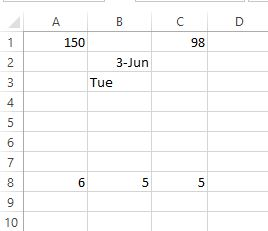A few weeks back I was asked to create a rule in Excel that would recalculate a cell’s value ONLY on Monday!  And store that calculation. What?  Hmm..  Then I thought, wait a minute, I should be able to do this in the cells directly. At first I tried to do this all in one cell and its got pretty messy. So instead I divided it up into two steps. First convert the date to string, then a simple if statement if the string = “Mon”.
To start, put a number in cell A1. Any number. place 6/2/2014 in B2. Place a number in A8 and B8. I have these lower for a reason you will see later.

So first the date. In cell B2 I have the following.
6/2/2014
In cell B3 I wrote this..
=TEXT(B2,”ddd”) – (in the real sheet, this cell is hidden somewhere.
Ok, now I do a simple =IF statement. For those of you who do not know, here is how a simple =IF statement works.. IF(logical_test,value_if_true,value_if_false)

So in cell C8 I have the following
=IF(B3=”Mon”, A8, B8)

B3 is the string value of the date from B2. A8 is the value if its Monday, and B8 is the value of its not. Done!

But there is a problem here… The actual goal is to have the new value STAY as the new value on and after Monday until the following Monday when it gets re calculated again. Ahhh. That is MUCH harder.

So here is where doing calculation in cells break down. Because we want to retain the new value after Monday, we cannot just have a simple =IF test because on tuesday, it goes back to the old value again. the IF statement has two conditions, true or false. We need to find a way to keep the new value we calculated on Monday throughout the week and then update it again the following Monday.

So VBScript to the rescue. If you have never written a script in Excel you may want to look up a few tutorials, its pretty powerful once you get into it, however, lets just walk you through it.

First right-click on the sheet tab in your spreadsheet. In my case, I right-clicked on ‘Sheet1’

A new window will popup called Microsoft Visual Basic for Applications.

Copy and paste the following code in. We will walk through it in a sec..

```Private Sub Worksheet_Change(ByVal Target As Range)
Dim Last As Integer
Application.EnableEvents = False

Dim currDateString As String
currDateString = Range("b3").Text
If currDateString = "Mon" Then Range("C1") = Range("A1")

Application.EnableEvents = True
End Sub
```

This is pretty much the same thing we did with the cells earlier, however, we are going to use the magic command ‘Application.EnableEvents = False’. What this does is still Excel not to calculate anything until told otherwise. Now, lets run our if statement. Again we have this broken up into two steps.

```Dim currDateString As String
currDateString = Range("b3").Text```

What these two lines do is set currDateString as a varible and then assign it the string value we already have in B3. If we wanted to be fancy we could have done this in code as well, but I’m trying to keep this simple.

Next we run our simple If statement.

`If currDateString = "Mon" Then Range("C1") = Range("A1")`

This is pretty stright forward, if currDateString (B3) = “Mon”, then have the cell C1 = A1.

And we are done. We turn on ‘Application.EnableEvents = True’ and let Excel do its thing.

Give it a try. Change A1 while the date is set to June 2 and you will see C1 updating. Change the date, and C1 no longer updates. Close the file and then reopen and the value are where you left off.

Neat eh?

I’m sure there are cooler ways to write this but again, I was going for simple here.

Enjoy!